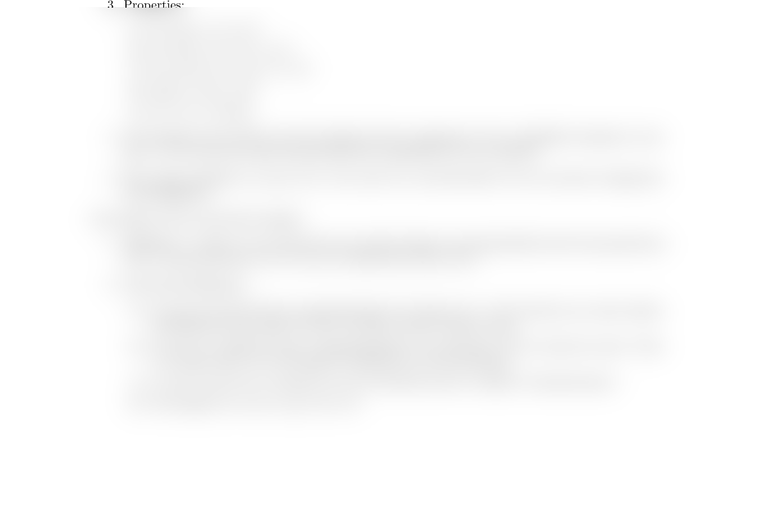# CSCI 2170 Lecture Notes - Lecture 12: Piecewise, Morphism Of Algebraic Varieties, Unit Vector

67 views2 pages
School
Course
ProfessorMath 241 Chapter 12 Dr. Justin O. Wyss-Gallifent
§12.1 Deﬁnitions and Examples of Vector Valued Functions
1. Deﬁnition: For each t,¯
F(t) (or usually, and later, ¯r(t)) points from the origin to a point on the
curve.
2. Classic examples: Circles, helices, lines and line segments, functions.
3. Properties: Without going too far into detail note that VVFs are vectors and so we can do
vectorish things with them like ¯
F×¯
Gand f¯
Fwhere fis a regular function.
§12.2 Limits and Continuity of VVFs
1. We can deﬁne limit the limit of a VVF by taking the limits of the components. We can then
deﬁne a VVF to be continuous iﬀ the components are continuous. We won’t go into detail but
it’s good to be aware that limits exist so that derivatives do.
§12.3 Derivatives and Integrals of VVFs
1. We can do this formally with limits but we won’t. In essence the derivative of a VVF is found by
taking the derivatives of the components.
2. Meaning: If ¯ris position then ¯v(t) = ¯r(t) is the velocity and is a vector which is tangent to the
curve, meaning it points in the direction of the curve. The function s(t) = ||¯v(t)|| is speed. The
vector ¯a(t) = ¯v(t) = ¯r′′(t) is acceleration and points to the inside of the curve.
3. Properties:
(a) ( ¯
F±¯
G)=¯
F±¯
G
(b) ( ¯
F·¯
G)=¯
F·¯
G+¯
F·¯
G
(c) ( ¯
F×¯
G)=¯
F×¯
G+¯
F×¯
G
(d) (f¯
F)=f¯
F+f¯
F
(e) ( ¯
Ff)=¯
F(f)f
4. The integral of the VVF is just the integral of the components. For an indenite integral we just
put a + ¯
Con the end rather than giving each component its own constant.
5. One classic problem is to give ¯a(t), ¯v(t0) and ¯r(t1) and then nd ¯r(t) by successive integration
and plugging-in.
§12.4 Space Curves and their Lengths
1. Denition: A (space) curve is just the curve itself, without worrying initially about the parametriza-
tion. A parametrization of Cis an ¯r(t) which draws that curve.
2. Associated denitions:
(a) A curve is closed if it has a parametrization ¯r(t) where ¯r(a) = ¯r(b) and the curve only touches
itself nitely many times at most. In other words it forms a loop.
(b) A curve is smooth if it has a parametrization ¯r(t) such that: If ¯r(t) exists for some tthen
¯r(t) exists and ¯r(t)6=¯
0 except its allowed to at the end points.
(c) A curve is piecewise smooth if it can be divided up into a number of smooth pieces.
(d) The length of a curve is Rb
a||¯r(t)|| dt.
Unlock document

This preview shows half of the first page of the document.
Unlock all 2 pages and 3 million more documents.